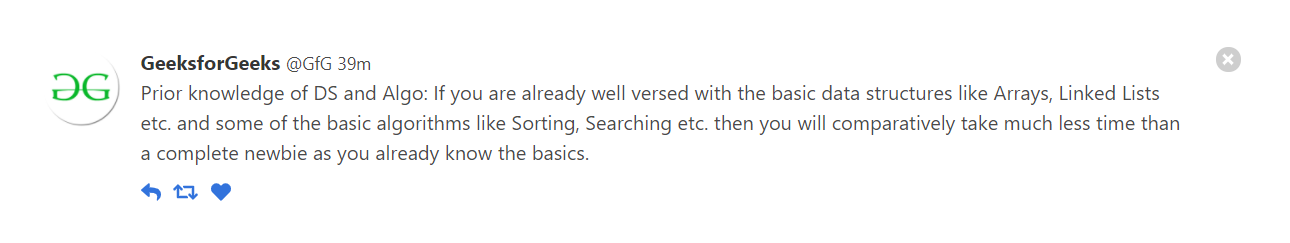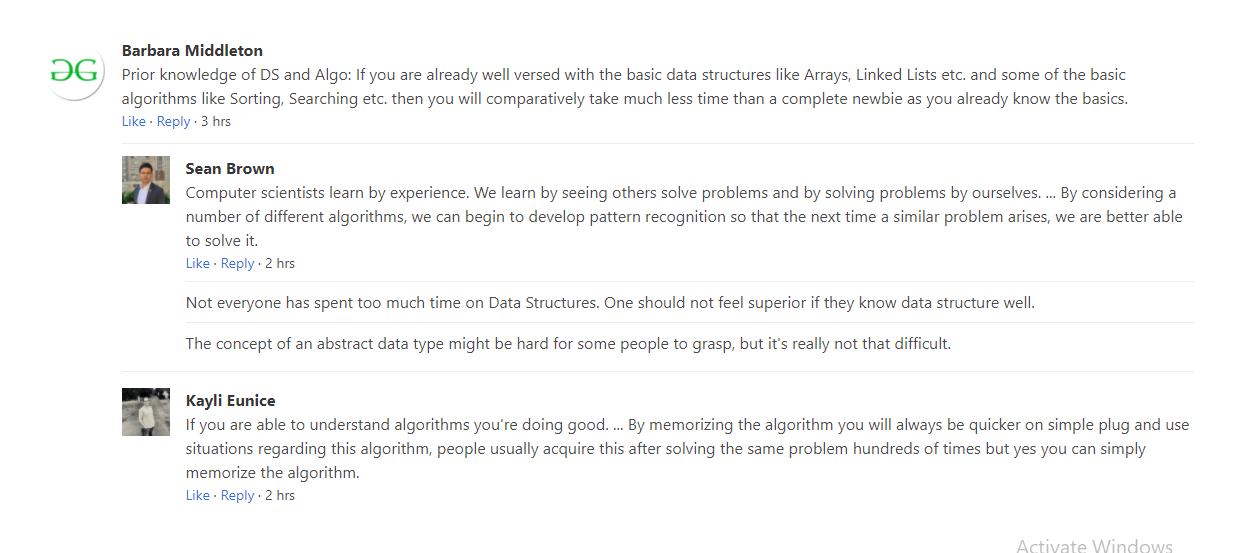GFG App
Open AppBrowser
Continue

Bulma is a free, open-source CSS framework based on Flexbox. It is component rich, compatible, and well documented. It is highly responsive in nature. It uses classes to implement its design.  The famous media object prevalent in social media interfaces, but it is useful in any context.

Example 1: This example illustrates simple social media interface using bulma media object.

 `<``html``> ` `  ``<``head``> ` `    ``<``title``>Bulma Media Object ` `    ``<``link` `rel``=``'stylesheet'`  `          ``href``= ` `'https://cdnjs.cloudflare.com/ajax/libs/bulma/0.7.5/css/bulma.css'``> ` `    ``` `    ``<``style``> ` `      ``div.adjust{ ` `        ``margin-top:50px; ` `      ``} ` ` `  `    `` ` `  `` ` `  ``<``body``>  ` `    ``<``div` `class``=``'container adjust'``> ` `      ``<``article` `class``=``"media"``> ` `        ``<``figure` `class``=``"media-left"``> ` `          ``<``p` `class``=``"image is-64x64"``> ` `            ``<``img` `src``= ` `"https://media.geeksforgeeks.org/wp-content/uploads/20200819145636/icon.png"``> ` `          `` ` `        `` ` `        ``<``div` `class``=``"media-content"``> ` `          ``<``div` `class``=``"content"``> ` `            ``<``p``> ` `              ``<``strong``>GeeksforGeeks  ` `            ``<``small``>@GfG <``small``>39m ` `              ``<``br``> ` `              ``Prior knowledge of DS and Algo:  ` `If you are already well versed with the  ` `              ``basic data structures like Arrays,  ` `Linked Lists etc. and some of the basic ` `              ``algorithms like Sorting, Searching etc.  ` `then you will comparatively take much  ` `              ``less time than a complete newbie  ` `as you already know the basics. ` `            `` ` `          `` ` `          ``<``nav` `class``=``"level is-mobile"``> ` `            ``<``div` `class``=``"level-left"``> ` `              ``<``a` `class``=``"level-item"``> ` `                ``<``span` `class``=``"icon is-small"``> ` `                  ``<``i` `class``=``"fas fa-reply"``> ` `              `` ` `              ``<``a` `class``=``"level-item"``> ` `                ``<``span` `class``=``"icon is-small"``> ` `                   ``<``i` `class``=``"fas fa-retweet"``> ` `              `` ` `              ``<``a` `class``=``"level-item"``> ` `                ``<``span` `class``=``"icon is-small"``> ` `                  ``<``i` `class``=``"fas fa-heart"``> ` `              `` ` `            `` ` `          `` ` `        `` ` `        ``<``div` `class``=``"media-right"``> ` `          ``<``button` `class``=``"delete"``> ` `        `` ` `      `` ` `    `` ` `  `` ` ``

Output:Example 2: We can include any Bulma elements like input, buttons, icons, textarea, etc. in this example we include Textarea element inside Bulma media object to illustrates how they work together.

 `<``html``> ` `  ``<``head``> ` `    ``<``title``>Bulma Media Object ` `    ``<``link` `rel``=``'stylesheet'`  `          ``href``= ` `'https://cdnjs.cloudflare.com/ajax/libs/bulma/0.7.5/css/bulma.css'``> ` `    ``` `    ``<``style``> ` `      ``div.adjust{ ` `        ``margin-top:50px; ` `      ``} ` ` `  `    `` ` `  `` ` `  ``<``body``> ` `    ``<``div` `class``=``'container adjust'``> ` `      ``<``article` `class``=``"media"``> ` `        ``<``figure` `class``=``"media-left"``> ` `          ``<``p` `class``=``"image is-64x64"``> ` `            ``<``img` `src``= ` `"https://media.geeksforgeeks.org/wp-content/uploads/20200819145636/icon.png"``> ` `          `` ` `        `` ` `        ``<``div` `class``=``"media-content"``> ` `          ``<``div` `class``=``"field"``> ` `            ``<``p` `class``=``"control"``> ` `              ``<``textarea` `class``=``"textarea"` `                   ``placeholder``=``"Ask your query..."``> ` `             `` ` `            `` ` `          `` ` `          ``<``nav` `class``=``"level"``> ` `            ``<``div` `class``=``"level-left"``> ` `              ``<``div` `class``=``"level-item"``> ` `                ``<``a` `class``=``"button is-info"``>Submit ` `              `` ` `            `` ` `          `` ` `        `` ` `      `` ` `    `` ` `  `` ` ``

Output:Textarea in media object

Example 3: This example illustrates nested media objects.

 `<``html``> ` `  ``<``head``> ` `    ``<``title``>Bulma Media Object ` `    ``<``link` `rel``=``'stylesheet'`  `          ``href``= ` `'https://cdnjs.cloudflare.com/ajax/libs/bulma/0.7.5/css/bulma.css'``> ` `    ``` `    ``<``style``> ` `      ``div.adjust{ ` `        ``margin-top:50px; ` `      ``} ` ` `  `    `` ` `  `` ` `  ``<``body``> ` `    ``<``div` `class``=``'container adjust'``> ` `      ``<``article` `class``=``"media"``> ` `        ``<``figure` `class``=``"media-left"``> ` `          ``<``p` `class``=``"image is-64x64"``> ` `            ``<``img` `src``= ` `"https://media.geeksforgeeks.org/wp-content/uploads/20200819145636/icon.png"``> ` `          `` ` `        `` ` `        ``<``div` `class``=``"media-content"``> ` `          ``<``div` `class``=``"content"``> ` `            ``<``p``> ` `              ``<``strong``>Barbara Middleton ` `              ``<``br``> ` `              ``Prior knowledge of DS and Algo:  ` `If you are already well versed with the basic data  ` `              ``structures like Arrays, Linked Lists etc. ` ` ``and some of the basic algorithms  ` `              ``like Sorting, Searching etc. ` ` ``then you will comparatively take much less ` `              ``time than a complete newbie  ` `as you already know the basics. ` `              ``<``br``> ` `<``small``><``a``>Like · <``a``>Reply · 3 hrs ` `            `` ` `          `` ` `       `  `          ``<``article` `class``=``"media"``> ` `            ``<``figure` `class``=``"media-left"``> ` `              ``<``p` `class``=``"image is-48x48"``> ` `                ``<``img` `src= ` `"https://media.geeksforgeeks.org/wp-content/uploads/20200819154857/ ` `                          ``7pitvZTX400x400-300x300.jpg"> ` `              `` ` `            `` ` `            ``<``div` `class``=``"media-content"``> ` `              ``<``div` `class``=``"content"``> ` `                ``<``p``> ` `                  ``<``strong``>Sean Brown ` `                  ``<``br``> ` `                  ``Computer scientists learn by experience. ` ` ``We learn by seeing others solve problems ` `                  ``and by solving problems by ourselves.  ` `... By considering a number of different  ` `                  ``algorithms, we can begin to develop ` ` ``pattern recognition so that  ` `                  ``the next time a similar problem  ` `arises, we are better able to solve it. ` `                  ``<``br``> ` `<``small``><``a``>Like · <``a``>Reply · 2 hrs ` `                `` ` `              `` ` `       `  `              ``<``article` `class``=``"media"``> ` `                ``Not everyone has spent too much time on Data Structures. ` ` ``One should not feel superior if they know data structure well. ` `              `` ` `       `  `              ``<``article` `class``=``"media"``> ` `                ``The concept of an abstract data type ` ` ``might be hard for some people to grasp,  ` `                ``but it's really not that difficult. ` `              `` ` `            `` ` `          `` ` `       `  `          ``<``article` `class``=``"media"``> ` `            ``<``figure` `class``=``"media-left"``> ` `              ``<``p` `class``=``"image is-48x48"``> ` `                ``<``img` `src= ` `"https://media.geeksforgeeks.org/wp-content/uploads/20200819154907 ` `                          ``/Z0pcI00C400x400-300x300.jpg"> ` `              `` ` `            `` ` `            ``<``div` `class``=``"media-content"``> ` `              ``<``div` `class``=``"content"``> ` `                ``<``p``> ` `                  ``<``strong``>Kayli Eunice ` `                  ``<``br``> ` `                  ``If you are able to understand  ` `algorithms you're doing good. ...  ` `                  ``By memorizing the algorithm you will always ` ` ``be quicker on simple plug and use ` `                  ``situations regarding this algorithm, ` ` ``people usually acquire this after solving  ` `                  ``the same problem hundreds of times  ` `but yes you can simply memorize the algorithm. ` `                  ``<``br``> ` `<``small``><``a``>Like · <``a``>Reply · 2 hrs ` `                `` ` `              `` ` `            `` ` `          `` ` `        `` ` `      `` ` `    `` ` `  `` ` ``

Output:Nested media object

My Personal Notes arrow_drop_up